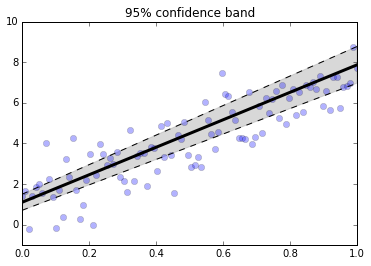In :
%matplotlib inline
%pylab inline
from scipy import stats
from IPython.html.widgets import interact

Populating the interactive namespace from numpy and matplotlib


## Regression¶

Regression means fitting data to a line or a polynomial. The simpliest case is a line where the model is the following:

$$y = a x + b + \epsilon$$

where $\epsilon \sim \mathcal{N}(0,\sigma^2)$ and we have to determine $a$ and $b$ from the data pairs $\lbrace (Y_i,X_i)\rbrace_{i=1}^n$. There is a subtle point here. The variable $x$ changes, but not as a random variable in this model. Thus, for fixed $x$, $y$ is a random variable generated by $\epsilon$. To be thorough, perhaps we should denote $\epsilon$ as $\epsilon_x$ to make this clear, but because $\epsilon$ is an identically-distributed random variable at each fixed $x$, we leave it out. Because of linearity and the Gaussian additive noise, the distribution of $y$ is completely characterized by its mean and variance.

$$\mathbb{E}(y) = a x + b$$$$\mathbb{V}(y) = \sigma^2$$

Using the maximum likelihood procedure we discussed earlier, we write out the log-likelihood function as

$$\mathcal{L}(a,b) = \sum_{i=1}^n \log \mathcal{N}(a X_i +b , \sigma^2) \propto \frac{1}{2 \sigma^2}\sum_{i=1}^n (Y_i-a X_i-b)^2$$

Note that I just threw out most of the terms that are irrelevent to the maximum-finding. Taking the derivative of this with respect to $a$ gives the following equation:

$$\frac{\partial \mathcal{L}(a,b)}{\partial a}= 2 \sum_{i=1}^n X_i (b+ a X_i -Y_i) =0$$

Likewise, we do the same for the $b$ parameter

$$\frac{\partial \mathcal{L}(a,b)}{\partial b}=2\sum_{i=1}^n (b+a X_i-Y_i) =0$$

Solving these two equations for $a$ and $b$ gives the parameters for the line we seek.

In :
a = 6;b = 1 # parameters to estimate
x = linspace(0,1,100)
# x = rand(100)*100 # x does not have to be monotone for this to work
y = a*x + np.random.randn(len(x)) +b

p,var_=np.polyfit(x,y,1,cov=True) # fit data to line
y_ = np.polyval(p,x) # estimated by linear regression

# draw comparative fits and hisogram of errors
fig,axs=subplots(1,2)
fig.set_size_inches((10,2))
ax =axs
ax.plot(x,y,'o',alpha=.5)
ax.plot(x,y_)
ax.set_xlabel("x",fontsize=18)
ax.set_ylabel("y",fontsize=18)
ax.set_title("linear regression; a =%3.3g\nb=%3.3g"%(p,p))
ax = axs
ax.hist(y_-y)
ax.set_xlabel(r"$\Delta y$",fontsize=18)
ax.set_title(r"$\hat{y}-y$",fontsize=18)
#ax.set_aspect(1/2)

Out:
<matplotlib.text.Text at 0xda558b0>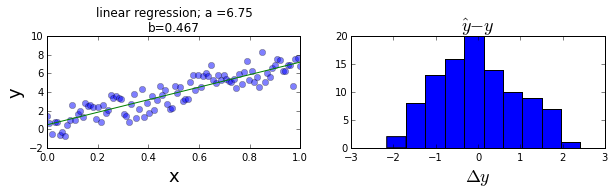The graph on the left shows the regression line plotted against the data. The estimated parameters are noted in the title. You can tweak the code in the cell above to try other values for $a$ and $b$ if you want. The histogram on the right shows the errors in the model. Note that the $x$ term does not have to be uniformly monotone. You can tweak the code above as indicated to try a uniform random distribution along the x-axis. It is also interesting to see how the above plots change with more or fewer data points.

## As separate tagged problems¶

We said earlier that we can fix the index and have separate problems of the form

$$y_i = a x_i +b + \epsilon$$

where $\epsilon \sim \mathcal{N}(0,\sigma^2)$. What could I do with just this one component of the problem? In other words, suppose we had k-samples of this component as in $\lbrace y_{i,k}\rbrace_{k=1}^m$. Following the usual procedure, we could obtain estimates of the mean of $y_i$ as

$$\hat{y_i} = \frac{1}{m}\sum_{k=1}^m y_{i,k}$$

However, this tells us nothing about the individual parameters $a$ and $b$ because they are not-separable in the terms that are computed, namely, we may have

$$\mathbb{E}(y_i) = a x_i +b$$

but we still only have one equation and the two unknowns. How about if we consider and fix another component $j$ as in

$$y_j = a x_j +b + \epsilon$$

and likewise, we have

$$\mathbb{E}(y_j) = a x_j +b$$

so at least now we have two equations and two unknowns and we know how to estimate the left hand sides of these equations from the data using the estimators $\hat{y_i}$ and $\hat{y_j}$. Let's see how this works in the code sample below.

In :
x0 =x
xn =x

y_0 = a*x0 + np.random.randn(20)+b
y_1 = a*xn + np.random.randn(20)+b

fig,ax=subplots()
ax.plot(x0*ones(len(y_0)),y_0,'o')
ax.plot(xn*ones(len(y_1)),y_1,'o')
ax.axis(xmin=-.02,xmax=1)

a_,b_=inv(np.matrix([[x0,1],[xn,1]])).dot(vstack([y_0,y_1]).mean(axis=1)).flat
x_array = np.array([x0,xn])
ax.plot(x_array,a_*x_array+b_,'-ro')
ax.plot(x,a*x+b,'k--');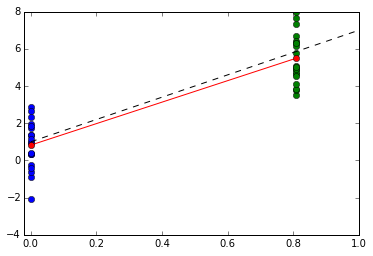In :
def plot_2_estimator(n=50):
x0 =x
xn =x[n]

y_0 = a*x0 + np.random.randn(20)+b
y_1 = a*xn + np.random.randn(20)+b

fig,ax=subplots()
ax.plot(x0*ones(len(y_0)),y_0,'o',alpha=.3)
ax.plot(xn*ones(len(y_1)),y_1,'o',alpha=.3)
ax.axis(xmin=-.25,xmax=1,ymax=10,ymin=-2)

a_,b_=inv(np.matrix([[x0,1],[xn,1]])).dot(vstack([y_0,y_1]).mean(axis=1)).flat
x_array = np.array([x0,x[-1]])
ax.grid()
ax.plot(x_array,a_*x_array+b_,'-ro')
ax.plot(x,a*x+b,'k--');

interact(plot_2_estimator,n=(1,99,1));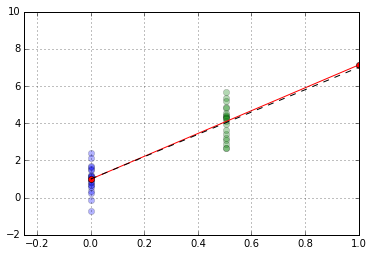We can write out the solution for the estimated parameters for this case where $x_0 =0$

$$\hat{a} = \frac{\hat{y_i} - \hat{y_0}}{x_i}$$$$\hat{b} = \hat{y_0}$$

The expectation of the first estimator is the following

$$\mathbb{E}(\hat{a}) = \frac{a x_i }{x_i}=a$$$$\mathbb{E}(\hat{b}) =b$$$$\mathbb{V}(\hat{a}) = \frac{2 \sigma^2}{x_i^2}$$$$\mathbb{V}(\hat{b}) = \sigma^2$$

The above results show that the estimator $\hat{a}$ has a variance that decreases as larger points $x_i$ are selected which is what we observed in the interactive graph above.

So, where are we now? We have a way of doing regression when there is only one sample at each $x_i$ point and we can do the same when we have many samples at each of two x-coordinates. Is there a way to combine the two approaches? This is something that is not considered in the usual discussions about regression, which always consider only the first case.

In this light, let us re-consider the first regression problem. We have only one sample at each $x_i$ coordinate and we want to somehow combine these into unbiased estimators of $a$ and $b$. The explicit formulas in this case are well known as the following:

$$\hat{a} = \frac{\mathbf{x}^T\mathbf{y}-(\sum X_i)(\sum Y_i)/n}{\mathbf{x}^T \mathbf{x} -(\sum X_i)^2/n}$$$$\hat{b} = \frac{-\mathbf{x}^T\mathbf{y}(\sum X_i)/n+\mathbf{x}^T \mathbf{x}(\sum Y_i)/n}{\mathbf{x}^T \mathbf{x} -(\sum X_i)^2/n}$$

Note that you can get the $\hat{b}$ from $\hat{a}$ by observing that for each component we have

$$Y_i = \hat{a} X_i + \hat{b}$$

and then by summing over the $n$ components we obtain

$$\sum_{i=1}^n Y_{i} = \hat{a} \sum_{i=1}^n X_i + n \hat{b}$$

Then, solving this equation for $\hat{b}$ with the $\hat{a}$ above gives the equations shown as below

$$\hat{b} = \frac{\sum_{i=1}^n Y_{i}-\hat{a} \sum_{i=1}^n X_i}{n}$$

In the case where there are only two $X_i$ values, namely $X_0=0$ and $X_j$, we obtain

$$\hat{a} = \frac{X_j \sum_{\lbrace X_j\rbrace} Y_i-m X_j \frac{\sum Y_i}{n}}{m X_j^2- m^2 X_j^2/n} = \frac{ \sum_{\lbrace X_j\rbrace} Y_i/m- \frac{\sum_{\lbrace X_0\rbrace} Y_i+\sum_{\lbrace X_j\rbrace} Y_i}{n}}{ X_j (1- m/n)}= \frac{\hat{y}_j-\hat{y}_0}{X_j}$$$$\hat{b} = \frac{\sum_{\lbrace X_0\rbrace} Y_i }{n-m} = \hat{y}_0$$

which is the same as our first estimator. This means that the general theory is capable of handling the case of multiple estimates at the same $X_j$. So what does this mean? We saw that having samples further out along the x-coordinate reduced the variance of the estimator in the two-sample study. Does this mean that in the regression model, where there is only one sample per x-coordinate, that those further along the x-coordinate are likewise more valuable in terms of variance reduction?

This is tricky to see in the above, but if we consider the simplified model without the y-intercept, we can obtain the following:

$$\hat{a} = \frac{\mathbf{x}^T \mathbf{y}}{\mathbf{x}^T \mathbf{x}}$$

with corresponding

$$\mathbb{V}(\hat{a}) = \frac{\sigma^2}{\|\mathbf{x}\|^2}$$

And now the relative value of large $\mathbf{x}$ is more explicit.

## Geometric View¶

In vector notation, we can write the following:

$$\mathbf{y} = a \mathbf{x} + b\mathbf{1} + \mathbf{\epsilon}$$

Then, by taking the inner-product with some $\mathbf{x}_1 \in \mathbf{1}^\perp$ we obtain

$$\langle \mathbf{y},\mathbf{x}_1 \rangle = a \langle \mathbf{x},\mathbf{x}_1 \rangle + \langle \mathbf{\epsilon},\mathbf{x}_1\rangle$$

and then sweeping the expectation over this (recall that $\mathbb{E}(\mathbf{\epsilon})=\mathbf{0}$)gives

$$\langle \mathbf{y},\mathbf{x}_1 \rangle = a \langle \mathbf{x},\mathbf{x}_1 \rangle$$

which we can finally solve for $a$ as

$$\hat{a} = \frac{\langle\mathbf{y},\mathbf{x}_1 \rangle}{\langle \mathbf{x},\mathbf{x}_1 \rangle}$$

that was pretty neat but now we have the mysterious $\mathbf{x}_1$ vector. Where does this come from? If we project $\mathbf{x}$ onto the $\mathbf{1}^\perp$, then we get the minimum-distance (in the $\mathbb{L}_2$ sense) approximation to $\mathbf{x}$ in the $\mathbf{1}^\perp$ space. Thus, we take

$$\mathbf{x}_1 = P_{\mathbf{1}^\perp} (\mathbf{x})$$

Remember that $P_{\mathbf{1}^\perp}$ is a projection matrix so the length of $\mathbf{x}_1$ is smaller than $\mathbf{x}$. This means that the denominator in the $\hat{a}$ equation above is really just the length of the $\mathbf{x}$ vector in the coordinate system of $P_{\mathbf{1}^\perp}$. Because the projection is orthogonal (namely, of minimum length), the Pythagorean theorem gives this length as the following:

$$\langle \mathbf{x},\mathbf{x}_1 \rangle ^2=\langle \mathbf{x},\mathbf{x} \rangle- \langle\mathbf{1}^T \mathbf{x} \rangle^2$$

The first term on the right is the length of the $\mathbf{x}$ vector and last term is the length of $\mathbf{x}$ in the coordinate system orthogonal to $P_{\mathbf{1}^\perp}$, namely that of $\mathbf{1}$. This is the same as the denominator of the $\hat{a}$ estimator we originally wrote. After all that work, we can use this geometric interpretation to understand what is going on in typical linear regression in much more detail. The fact that the denominator is the orthogonal projection of $\mathbf{x}$ tells us that this is the choice of $\mathbf{x}_1$ that has the strongest effect (i.e. largest value) on reducing the variance of $\hat{a}$. This is because it is the term that enters in the denominator which, as we observed earlier, is what is reducing the variance of $\hat{a}$. We already know that $\hat{a}$ is an unbiased estimator and because of this we know that it is additional of minimum variance. Such estimators are know and minimum-variance unbiased estimators (MVUE).

In the same spirit, let's examine the numerator of $\hat{a}$. We can write $\mathbf{x}_{1}$ as the following

$$\mathbf{x}_{1} = \mathbf{x} - P_{\mathbf{1}} \mathbf{x}$$

where $P_{\mathbf{1}}$ is projection matrix of $\mathbf{x}$ onto the $\mathbf{1}$ vector. Using this, the numerator of $\hat{a}$ becomes

$$\langle \mathbf{y}, \mathbf{x}_1\rangle =\langle \mathbf{y}, \mathbf{x}\rangle -\langle \mathbf{y}, P_{\mathbf{1}} \mathbf{x}\rangle$$

Note that is the outer product of $\mathbf{1}$ as in the following:

$$P_{\mathbf{1}} = \mathbf{1} \mathbf{1}^T \frac{1}{n}$$

so that writing this out explicitly gives

$$\langle \mathbf{y}, P_{\mathbf{1}} \mathbf{x}\rangle = \left(\mathbf{y}^T \mathbf{1}\right) \left( \mathbf{1}^T \mathbf{x}\right)$$

which, outside of a $\frac{1}{n}$ scale factor I am omitting to reduce notational noise, is the same as

$$\left(\sum Y_i\right)\left(\sum X_{i}\right)/n$$

So, plugging all of this together gives what we have seen before as

$$\hat{a} \propto \mathbf{y}^T \mathbf{x} - \left(\sum Y_i\right)\left(\sum X_{i}\right)/n$$

The variance of $\hat{a}$ is the following:

$$\mathbb{V}(\hat{a}) = \sigma^2 \frac{\|\mathbf{x}_1\|^2}{\langle\mathbf{x},\mathbf{x}_1\rangle^2}$$

Doing the exact same thing with $\hat{b}$ gives

$$\hat{b} = \frac{\langle \mathbf{y},\mathbf{x}^{\perp} \rangle}{\langle \mathbf{1},\mathbf{x}^{\perp} \rangle}= \frac{\langle \mathbf{y},\mathbf{1}-P_{\mathbf{x}}(\mathbf{1})\rangle}{\langle \mathbf{1},\mathbf{1}-P_{\mathbf{x}}(\mathbf{1}) \rangle}$$

where

$$P_{\mathbf{x}} = \frac{\mathbf{\mathbf{x} \mathbf{x}^T}}{\| \mathbf{x} \|^2}$$

This is unbiased with variance

$$\mathbb{V}(\hat{b}) = \sigma^2 \frac{\langle \mathbf{\xi},\mathbf{\xi}\rangle}{\langle \mathbf{1},\mathbf{\xi}\rangle^2}$$

where $$\mathbf{\xi} = \mathbf{1} - P_{\mathbf{x}} (\mathbf{1})$$

## Regularized Linear Regression¶

Now, after moving all of that machinery, we should have a very clear understanding of what is going on in every step of the linear regression equation. The payoff for all this work is that now we know where to intercede in the construction to satisfy other requirements we might have to deal with real-world data problems.

In :
n = len(x)
one = ones((n,))

P_1 = ones((n,n))/n-eye(n)*1.35
#P_1 = ones((n,n))/n
P_x = outer(x,x)/dot(x,x)-eye(n)*1.3
x_1 = x-dot(P_1,x)
sumx = sum(x)
o=[]
ofit=[]
for i in range(500):
y = a*x + np.random.randn(n)+b
a_hat = dot(x_1,y)/dot(x_1,x)
b_hat = dot(y,one-dot(P_x,one))/dot(one,one-dot(P_x,one))
o.append((a_hat,b_hat))
ofit.append(tuple(polyfit(x,y,1)))

ofit = array(ofit)
o = array(o)

fig,axs=subplots(2,2)
fig.set_size_inches((6,5))
ax=axs[0,0]
ax.hist(o[:,0],20,alpha=.3)
ax.hist(ofit[:,0],20,alpha=.3)
ax=axs[0,1]
ax.plot(o[:,0],ofit[:,0],'.')
ax.plot(linspace(4,10,2),linspace(4,10,2))
ax.set_aspect(1)
ax.set_title('var=%3.3g vs. %3.3g'%(var(o[:,0]),var(ofit[:,0])))
ax=axs[1,0]
ax.hist(o[:,0],20,alpha=.3)
ax.hist(ofit[:,0],20,alpha=.3)
ax=axs[1,1]
ax.plot(o[:,1],ofit[:,1],'.')
ax.plot(linspace(0,1,3),linspace(0,1,3))
ax.set_aspect(1)
ax.set_title('var=%3.3g vs. %3.3g'%(var(o[:,1]),var(ofit[:,1])))

Out:
<matplotlib.text.Text at 0xd9e0630>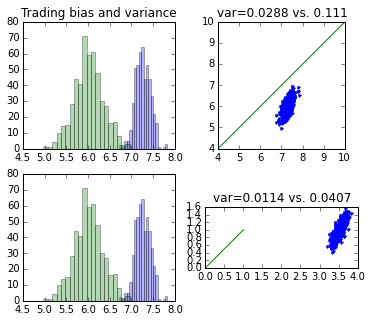In :
one = ones((n,))
xi=one-dot(P_x,one)
(a_,b_),var_= polyfit(x,y,1,cov=True)
sigma2_est = var(polyval([a_,b_],x)-y)

b_hat_var = sigma2_est*dot(xi,xi)/dot(one,xi)**2
a_hat_var = sigma2_est*dot(x_1,x_1)/dot(x_1,x)**2
a_hat_lo,a_hat_hi=stats.norm(a_hat,sqrt(a_hat_var)).interval(.95)
b_hat_lo,b_hat_hi=stats.norm(b_hat,sqrt(b_hat_var)).interval(.95)

plot(x,y,'o',alpha=.5,ms=5.,lw=4.,label='data')
plot(x,polyval([a_hat,b_hat],x),lw=4.,label='regularized',alpha=.5)
plot(x,polyval([a,b],x),lw=3.,label='true',alpha=.5)
plot(x,polyval(polyfit(x,y,1),x),lw=3.,label='MVUE',alpha=.5,color='k')
plot(x,polyval([a_hat_hi,b_hat_hi],x),'--c')
plot(x,polyval([a_hat_lo,b_hat_lo],x),'--c')
legend(loc=0);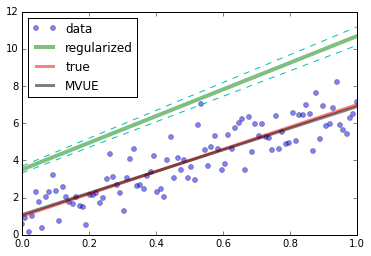In :
x = linspace(0,1,n)
sumx=sum(x)
y = a*x + np.random.randn(n)+b
sumy = sum(y)
def plot_lin_regularizer(lam= 0.1):
P_1 = ones((n,n))/n-eye(n)*lam
x_1 = x-dot(P_1,x)
a_hat = dot(x_1,y)/dot(x_1,x)
b_hat = (sumy - a_hat*(sumx))/n
y_hat = polyval([a_hat,b_hat],x)
plot(x,y,'o',alpha=.5,ms=5.,lw=4.,label='data')
plot(x,y_hat,lw=4.,label='regularized',alpha=.5)
title(r'$\lambda$ = %3.3g;MSE=%3.2g,ahat=%3.2f'%(lam,linalg.norm(y_hat-y)**2,
a_hat),fontsize=14)
plot(x,polyval([a,b],x),lw=3.,label='true',alpha=.5)
plot(x,polyval(polyfit(x,y,1),x),lw=3.,label='MVUE',alpha=.5,color='k')
legend(loc=0)
axis((0,1,0,10))
interact(plot_lin_regularizer,lam=(-1.,3.,.05))

Out:
<function __main__.plot_lin_regularizer>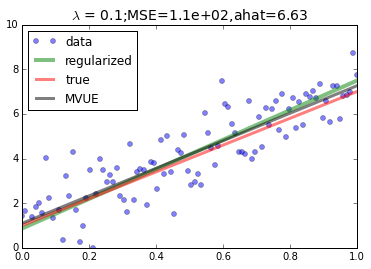## Multi-dimensional Gaussian Model¶

Although the procedure for estimating the parameters is straightforward, we can approach it from another angle to get more insight using a multi-dimensional Gaussian model. We denote the vector of the set of $\lbrace Y_i\rbrace$ as $\mathbf{y}$ and likewise for $\mathbf{x}$. This means we can write the multi-dimensional Gaussian model as $\mathcal{N}(a \mathbf{x}+ b \mathbf{I},\mathbf{I}\sigma^2)$ where $\mathbf{I}$ indicates the identity matrix.

In :
def lin_regress(x,y,lam=0,kap=0,alpha=0.95):
'linear regression with optional regularization'
n = len(x)
sumx = sum(x)
sumy = sum(y)
one = ones((n,))
P_1 = ones((n,n))/n-eye(n)*lam
P_x = outer(x,x)/dot(x,x)-eye(n)*kap
xi=one-dot(P_x,one)
x_1 = x-dot(P_1,x)
a_hat = dot(x_1,y)/dot(x_1,x)
b_hat = dot(y,one-dot(P_x,one))/dot(one,one-dot(P_x,one))
(a_,b_)= polyfit(x,y,1)
sigma2_est = var(polyval([a_,b_],x)-y) # OLS for noise estimate
b_hat_var = sigma2_est*dot(xi,xi)/dot(one,xi)**2
a_hat_var = sigma2_est*dot(x_1,x_1)/dot(x_1,x)**2
a_hat_lo,a_hat_hi=stats.norm(a_hat,sqrt(a_hat_var)).interval(alpha)
b_hat_lo,b_hat_hi=stats.norm(b_hat,sqrt(b_hat_var)).interval(alpha)
return (a_hat,b_hat,a_hat_hi-a_hat_lo,b_hat_hi-b_hat_lo)

In :
def plot_lin_regularizer_band(lam= 0.0,kap=0.0):
fig,ax = subplots()
ax.plot(x,y,'o',alpha=.3)
ax.plot(x,polyval([a_hat,b_hat],x),color='k',lw=3.)